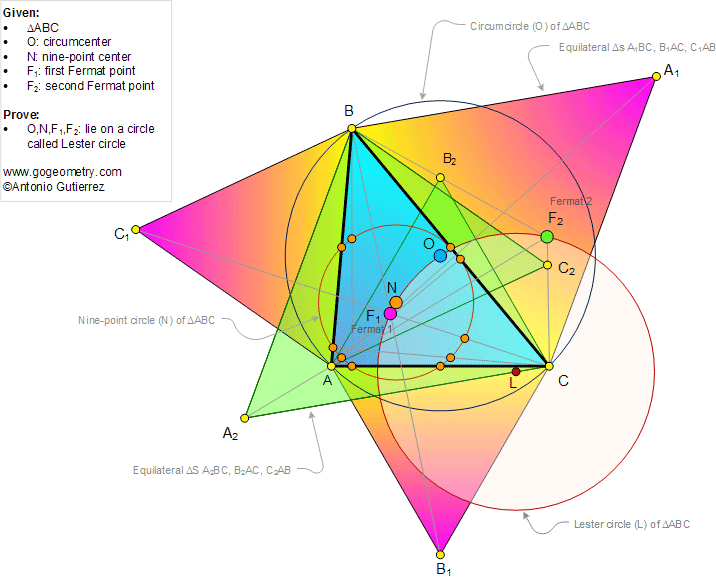###### Geometry Problem 905: Lester Circle Theorem, Triangle, Circumcenter, Nine-Point Center, First and Second Fermat Point. Levels: School, College, Mathematics Education

< PREVIOUS PROBLEM  |  NEXT PROBLEM >Given a triangle ABC (see figure above), the circumcenter O, Nine-point center N, First F1 and Second F2 Fermat points lie on a circle, called Lester circle.

This theorem was discovered by professor June A, Lester in 1996. Reference: June A. Lester, "Triangles III: Complex triangle functions", Aequationes Mathematicae, volume 53, pages 4–35, 1997.

Home | SearchGeometry | Problems | All Problems | Open Problems | Visual Index | 10 Problems | Problems Art Gallery Art | 901-910 | Triangles | Circle | Center | Circumcenter | Cyclic Quadrilateral | Concyclic Points | Equilateral First Fermat Point | Second Fermat Point | Email | Solution/Comment | by Antonio Gutierrez